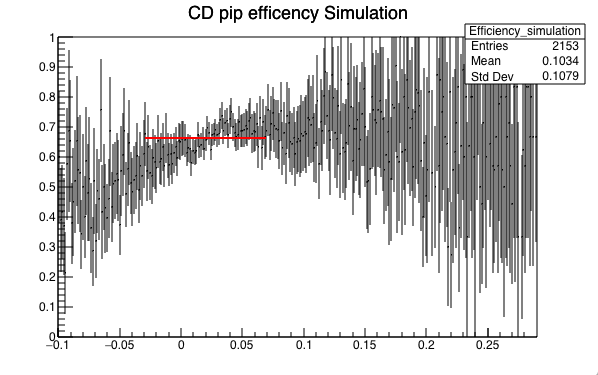# Error of a fit

Hello Rooters,

I have a straight line fit(see attached plot red line).This is the Average value of my efficiency,
I want to take the error of this fit.
There is only one parameter.

``
TF1 *fAve_simulation = new TF1(“fAve_simulation”,"",-0.03,0.07);
fAve_simulation->SetParameter(0,0.25);
Efficiency_simulation->Fit(“fAve_simulation”,"","",-0.03,0.07);
fAve_simulation->SetLineColor(2);
fAve_simulation->Draw(“sames”);

``If there are two parameters what could be the error of that fit?
Thanks
Dilini

`for (Int_t i = 0; i < fAve_simulation->GetNpar(); i++) std::cout << fAve_simulation->GetParName(i) << " = " << fAve_simulation->GetParameter(i) << " +- " << fAve_simulation->GetParError(i) << "\n"`

BTW. Before fitting, try to add: `gStyle->SetOptFit();`

Thank You Wile_E_Coyote.
Is there a way to draw these error bars in the plot?

fAve_simulation->Draw(" E3 same"); doesn’t work.

Thanks
Dil

Thanks
Dilini

There is no direct way to draw “confidence bands” for functions in ROOT.

Thank you.

Different question.
Can we use covarianceMatrix to calculate the error of 2 parameter fit?

TFitResultPtr r = Efficiency_simulation->Fit(“fAve_simulation”,"","",0.78,0.98);
TMatrixD cov = r->GetCorrelationMatrix();
TMatrixD cor = r->GetCovarianceMatrix();
cov.Print();
cor.Print();

The above gave me an error.I mean crash…

Thanks
Dil

`TFitResultPtr r = Efficiency_simulation->Fit(fAve_simulation, "S", "", 0.78, 0.98);`

This topic was automatically closed 14 days after the last reply. New replies are no longer allowed.